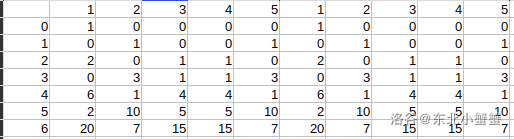# 传球游戏

## 输入输出格式

### 输出格式

$1$个整数，表示符合题意的方法数。

## 输入输出样例

### 输入样例 #1

3 3


### 输出样例 #1

2


## 说明

40%的数据满足：$3 \le n \le 30,1 \le m \le 20$
100%的数据满足：$3 \le n \le 30,1 \le m \le 30$

2008普及组第三题

# 分析$dp_{i,j}=\begin{cases}1&i = 0, j = 1\\0&i = 0\\dp_{i - 1, n} + dp_{i - 1, 2}&j = 1\\dp_{i - 1, n - 1} + dp_{i - 1, 1}&j = n\\dp_{i - 1, j - 1} + dp_{i - 1, j + 1}\end{cases}$

=IF(COLUMN()<=6,IF(COLUMN()<>2,INDIRECT(ADDRESS(ROW()-1,COLUMN()-1))+INDIRECT(ADDRESS(ROW()-1,COLUMN()+1)),INDIRECT(ADDRESS(ROW()-1,COLUMN()+1))+INDIRECT(ADDRESS(ROW()-1,6))),INDIRECT(ADDRESS(ROW(),COLUMN()-5)))

# 代码

/*
* @Author: crab-in-the-northeast
* @Date: 2020-03-03 21:36:03
* @Last Modified by: crab-in-the-northeast
* @Last Modified time: 2020-03-03 23:12:37
*/
#include <iostream>
#include <cstdio>

const int maxn = 35;
int dp[maxn][maxn];

int main() {
int n, m;
scanf("%d%d",&n,&m);
dp = 1;
for(int i = 1; i <= m; i++) {
dp[i] = dp[i - 1][n] + dp[i - 1];
dp[i][n] = dp[i - 1][n - 1] + dp[i - 1];

for(int j = 2; j <= n - 1; j++)
dp[i][j] = dp[i - 1][j - 1] + dp[i - 1][j + 1];

}
printf("%d\n",dp[m]);
return 0;
}


# 评测结果

AC 100R31341950

posted @ 2020-03-03 23:27  东北小蟹蟹  阅读(183)  评论(0编辑  收藏  举报